# 求垂心 (Orthocentre)坐標的方法

• 03/12/2014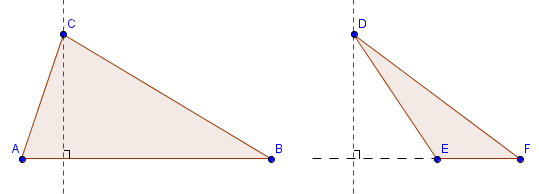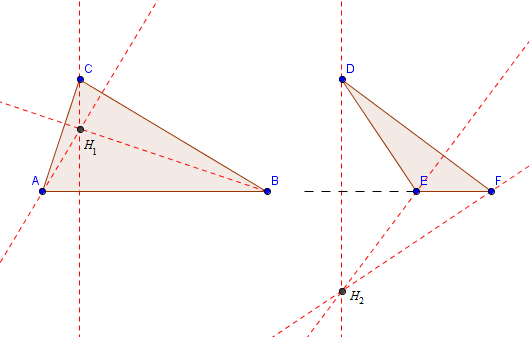$$m_{\small CH} \cdot m_{\small AB} = -1$$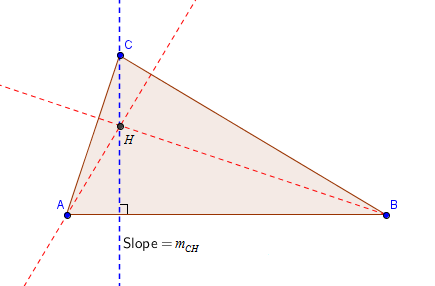$$\begin{cases} m_{\small CH} \cdot m_{\small AB} = -1 \\ m_{\small BH} \cdot m_{\small AC} = -1 \\ m_{\small AH} \cdot m_{\small BC} = -1 \end{cases}$$

\begin{align*} m_{\small AH} \cdot m_{\small BC} &= -1\\ \frac{k-1}{h-2} \cdot \frac{4-2}{4-6} &= -1\\ \frac{k-1}{h-2} \cdot \frac{2}{-2} &= -1\\ \frac{k-1}{h-2} \cdot (-1) &= -1\\ \frac{k-1}{h-2} &= 1\\ k-1 &= h-2\\ h &= k+1 …. (1) \end{align*}

\begin{align*} m_{\small BH} \cdot m_{\small AC} &= -1\\ \frac{k-2}{h-6} \cdot \frac{4-1}{4-2} &= -1\\ \frac{k-2}{h-6} \cdot \frac{3}{2} &= -1\\ \frac{k-2}{h-6} &= \frac{-2}{3}\\ 3k-6 &= -2h +12\\ 2h+3k &= 18 …. (2) \end{align*}

$$h=\frac{21}{5},\ k = \frac{16}{5}$$

∴ 垂心的坐標 = $\large (\frac{21}{5},\frac{16}{5})$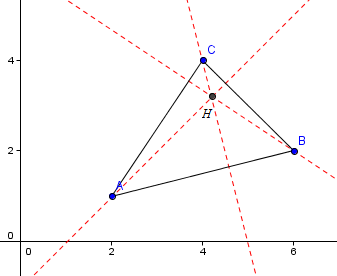1. 如果該三角形是鈍角三角形，這方法同樣適用。
2. 如果三角形的其中一條邊是垂直線，該邊的斜率是無定義(undefined)；同樣如其中一條邊是水平線，其斜率是零。在這兩個情況下，均無法建立其相關的方程。因此須要運用另外兩條方程來求取答案。
3. 如下圖所示，該三角形同時擁有垂直線和水平線，應怎樣處理? 答案其實很簡單， A點就是該三角形的垂心。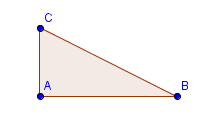H(h, k)為三角形的垂心(Orthocentre)

$$\begin{cases} m_{\small CH} \cdot m_{\small AB} = -1 \\ m_{\small BH} \cdot m_{\small AC} = -1 \\ m_{\small AH} \cdot m_{\small BC} = -1 \end{cases}$$

• 2021P2Q41
• 2018P2Q40
• 2015P2Q42
• 2012練習卷P2Q42
• 2008P2Q52

### 回應 (2)

1.ycm 說：

\begin{align*}
m_{\small AH} \cdot m_{\small BC} &= -1\\
\frac{k-1}{h-2} \cdot \frac{4-2}{4-6} &= -1\\
\frac{k-1}{h-2} \cdot \frac{2}{-2} &= -1\\
\frac{k-1}{h-2} \cdot (-1) &= -1\\
\frac{k-1}{h-2} &= 1\\
k-1 &= h-2\\
h &= k+1 …. (1)
\end{align*}

ur coding is unfinsh??

•Thomas Fok 說：

Does it work now? I found some mistakes in the code but it worked ok on Chrome and Firefox. So, I cannot notice the problem. May I ask which browser you are using?